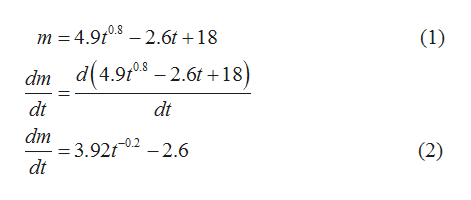# Water is poured into a container that has a leak. The mass m of the water is given as a function of time t by m = 4.9t0.8 - 2.6t + 18, with t ≥ 0, m in grams, and t in seconds. (a) At what time is the water mass greatest, and (b) what is that greatest mass? What is the rate of mass change at (c) t = 1.9 s and (d) t = 4.9 s?

Question
21 views

Water is poured into a container that has a leak. The mass m of the water is given as a function of time t by m = 4.9t0.8 - 2.6t + 18, with t ≥ 0, m in grams, and t in seconds. (a) At what time is the water mass greatest, and (b) what is that greatest mass? What is the rate of mass change at (c) t = 1.9 s and (d) t = 4.9 s?

check_circle

Step 1

Write the given function of time for the mass of the water, and differentiate with respect to time.help_outlineImage Transcriptionclosem 4.9t.8 2.6t +18 (1) dm d(4.9f08 -2.6t +18) dt dt dm = 3.92f02 -2.6 dt (2) fullscreen
Step 2

(a)

The water mass will be greatest when the rate of mass change will be equal to zero. Thus, time for the greatest water mass can be calculated as,

Step 3

(b)

Substitute the value of time for the greatest water mass in the given ...

### Want to see the full answer?

See Solution

#### Want to see this answer and more?

Solutions are written by subject experts who are available 24/7. Questions are typically answered within 1 hour.*

See Solution
*Response times may vary by subject and question.
Tagged in

### Other